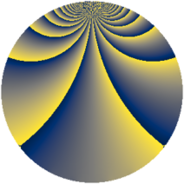# Properties

 Label 927.1.vLevel $927$ Weight $1$ Character orbit 927.v Rep. character $\chi_{927}(10,\cdot)$ Character field $\Q(\zeta_{34})$ Dimension $16$ Newform subspaces $1$ Sturm bound $104$ Trace bound $0$

# Related objects

## Defining parameters

 Level: $$N$$ $$=$$ $$927 = 3^{2} \cdot 103$$ Weight: $$k$$ $$=$$ $$1$$ Character orbit: $$[\chi]$$ $$=$$ 927.v (of order $$34$$ and degree $$16$$) Character conductor: $$\operatorname{cond}(\chi)$$ $$=$$ $$103$$ Character field: $$\Q(\zeta_{34})$$ Newform subspaces: $$1$$ Sturm bound: $$104$$ Trace bound: $$0$$

## Dimensions

The following table gives the dimensions of various subspaces of $$M_{1}(927, [\chi])$$.

Total New Old
Modular forms 80 32 48
Cusp forms 16 16 0
Eisenstein series 64 16 48

The following table gives the dimensions of subspaces with specified projective image type.

$$D_n$$ $$A_4$$ $$S_4$$ $$A_5$$
Dimension 16 0 0 0

## Trace form

 $$16q + q^{4} - 2q^{7} + O(q^{10})$$ $$16q + q^{4} - 2q^{7} + 2q^{13} - q^{16} - 2q^{19} - q^{25} + 2q^{28} - 3q^{49} - 2q^{52} + 2q^{61} + q^{64} + 2q^{76} + 2q^{79} - 13q^{91} - 15q^{97} + O(q^{100})$$

## Decomposition of $$S_{1}^{\mathrm{new}}(927, [\chi])$$ into newform subspaces

Label Dim. $$A$$ Field Image CM RM Traces $q$-expansion
$$a_2$$ $$a_3$$ $$a_5$$ $$a_7$$
927.1.v.a $$16$$ $$0.463$$ $$\Q(\zeta_{34})$$ $$D_{34}$$ $$\Q(\sqrt{-3})$$ None $$0$$ $$0$$ $$0$$ $$-2$$ $$q+\zeta_{34}^{11}q^{4}+(\zeta_{34}^{2}+\zeta_{34}^{16})q^{7}+\cdots$$